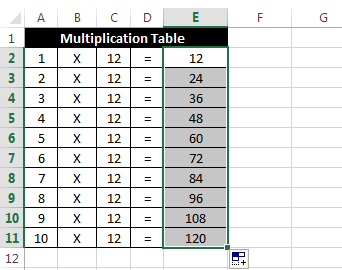# Excel Relative and Absolute References – Explained

In our last post about Finding duplicates in Excel, we created a clever formula to find only single instance of a duplicate value using the concept of Excel absolute references. In that post we were unable to discuss further about Absolute and Relative references, but today we will understand what these references mean.

### Excel Relative References:

Relative reference formulas are the most basic and widely used type of formulas. In this type of referencing we don’t use ‘\$’ in-front of the references. Here, when we use auto-fill to enter the formula automatically in a range, then Excel recognizes a pattern in the formula and fills the formula as per that pattern.

Let’s understand this with an example:

In the below example we have to calculate a Multiplication table in Excel.So, for the calculating the first item the formula would be: `=A2*C2`

Now, if you will drag this formula to the entire range then excel will automatically adjust the references in a correct way.So, how this happens?

Actually when you enter the first formula as `=A2*C2` excel recognizes a pattern i.e. Four cells to the left of current cell multiplied by 2 cells to the left of current cell. Now, when you drag this formula to the end then excel fills the formula according to the previous pattern.

This is called Relative Referencing because the pattern is dependent on the current cell.

### Excel Absolute References:

Absolute reference formulas are generally used less often as compared to relative reference formulas. Here, we do not want Excel to fill the formula using some pattern and hence we hold certain rows, columns or cells as constant reference (which should not be changed). This is done by using a ‘\$’ sign before the reference.

 \$A1 This allows the row reference to change, but the column reference will be constant. A\$1 This allows the column reference to change, but the row reference will be constant. \$A\$1 Both the Row as well as column references are constant.

Now, many people would argue that relative references are better because they follow a pattern and hence are more flexible. But there are few situations where relative references cannot be used and in such places we use absolute references.

Let’s understand this with an Example:

In the below image we have to create a multiplication table array.Now, in the first cell obviously the formula would be `=B4*A5`

This formula is right for the first cell (i.e. B5) as it results into 1.But if you drag this formula to the end then you will see some strange results as shown below.So, why this happens?

As I have foretold that excel recognizes a pattern in the formulas and then fills the same pattern in the other cells when you drag the formula. In this case same thing happens, when you write the formula for first cell (i.e. B5), your formula is: `=B4*A5`

Excel recognizes this as a pattern: One cell on top of current cell multiplied by One cell to the left of current cell.

But this is not what you want Excel to do, so you will use Absolute References in order to lock the references.In the above image I have used a formula comprising of absolute references to calculate the results. The formula is: `=B\$4*\$A5`

Now, if you drag this formula you will see that it always gives the correct result.

When you drag this formula `(=B\$4*\$A5)`. The first part i.e. B\$4 holds the row reference constant but column reference can change i.e. this means it will always point to the cells in the yellow row. The second part i.e. (\$A5) holds the column reference constant but row reference can change, this makes sure that the second part in the formula always points to cells in the green row. And hence it gives correct result.

This is called Absolute Referencing.

### Bonus Tip:

Pressing F4 key while writing a formula makes the references absolute. Repeatedly pressing ‘F4’ key results into cycling through all the possible types of absolute references for the current formula.

So, this was all about Excel Relative and Absolute References.About Ankit Kaul

Ankit is the founder of Excel Trick. He is tech Geek who loves to sit in front of his square headed girlfriend (his PC) all day long. :D. Ankit has a strong passion for learning Microsoft Excel. His only aim is to turn you guys into 'Excel Geeks'.